## Unit 5 Study Guide: States of Matter

### 5a: Use kinetic-molecular theory to explain the relationships among gas volume, temperature, number of moles, and pressure.

Chemists use the Kinetic Molecular Theory of Gases to explain the behavior of gases on a macroscopic (visible to the naked eye) scale.

• Describe the kinetic molecular theory of gases.
• Define the pressure of a gas.
• Explain how the Kinetic Molecular Theory of Gases gives relationships among volume, temperature, number of moles of gas, and pressure.

The Kinetic Molecular Theory of Gases describes how gases behave and can allow us to predict certain properties of gases.

Review the basic rules in Section 1: The Basic Idea of Kinetic Molecular Theory, in Gas Molecules in Motion.

Here are the five parts of the Kinetic Molecular Theory of Gases:

1. Gas particles are in constant motion and separated from each other by large distances. Therefore, the volume of the gas molecules in a sample is extremely small compared to the overall volume of the gas. Most of the volume of a gas is empty space.
2. Molecules of an ideal gas do not interact with each other or the walls of the container.
3. Molecules of a gas are in constant random motion. This random motion is in straight lines.
4. All collisions among gas molecules are elastic collisions. This means that there is no energy loss when gas molecules collide.
5. The temperature of a gas is directly proportional to its average kinetic energy, or energy of motion.

Particles in a gas phase are in constant motion and fill the entire container they inhabit. The gas particles are constantly hitting the surface of the container they are in, and exert force on the container surface. We define the pressure of the gas as the amount of force the gas exerts on the container they inhabit per unit area.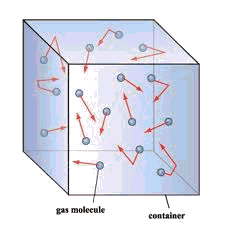The Kinetic Molecular Theory of Gases explains basic properties of gases.

Review Section 2: How Kinetic Molecular Theory Explains Gas Laws, in Gas Molecules in Motion.

According to the Kinetic Molecular Theory of Gases, gas must have pressure because it is constantly in motion in its container. When gas particles hit the wall of the container, they exert a force. Force divided by area is defined as pressure.

We can express the kinetic energy, the energy of motion, mathematically as:(ke represents kinetic energy, m represents mass, v represents velocity).

Keep in mind that temperature corresponds directly with average kinetic energy. As temperature rises, the velocity (speed) of the gas particles, as per the equation above, also increases.

The pressure of a gas also corresponds directly with its kinetic energy. As kinetic energy rises, the force with which the gas particles hit the wall of the container increases. Consequently the pressure also increases.

As we increase the number of moles of gas in a container at constant pressure, more gas particles will hit the walls of the container in a given amount of time. If the pressure is constant, this will force the volume of the container to increase.

### 5b: Perform dimensional analysis conversions for gas law calculations.

Many gas laws describe the behavior of gases under specific sets of conditions. These mathematical relations allow us to perform calculations—to calculate properties, such as pressure, temperature, volume, and number of moles of a gas under given conditions.

• Use the gas constant with proper units to complete gas law equation calculations.
• Convert units given in a question to units consistent with the units of the gas constant.

We express the gas constant mathematically as:

R = 0.082 L atm/mol K

Keep in mind that for gas law calculations:

• Measurements of volume must be in L (liters);
• Measurements of pressure must be in atm (atmospheres);
• Measurements of substance must be in moles; and
• Measurements of temperature must be in K (the Kelvin temperature scale).

Review the Ideal Gas Law in Section 4: The Ideal Gas Equation of State, in The Basic Gas Laws. See Problem Example 3 for an example of how to use the Ideal Gas Law.

### 5c: State the ideal gas law.

The ideal gas law shows the relationship among pressure, temperature, volume, and number of moles of a gas in ideal conditions.

• State the ideal gas law.

We express the ideal gas law mathematically as:

PV = nRT

(P represents pressure in atm, or atmospheres; V represents volume in L, or liters; n represents the number of moles; R represents the gas constant, or 0.082 L atm/mol K; and T represents temperature, which is K or the Kelvin scale.)

Review the Ideal Gas Law in Section 4: The Ideal Gas Equation of State, in The Basic Gas Laws. See Problem Example 3 for an example of how to use the Ideal Gas Law.

### 5d: Describe the motion of particles in liquids and the properties of liquids.

Liquids exist between a substance’s melting and boiling point.

• Describe the properties of a liquid.
• How do particles in a liquid move?

Liquids are mobile, or exhibit mobility, which means their molecules can move around and change shape according to the container they inhabit. Viscosity describes another property of liquids, which means their resistance to flow. A liquid with high viscosity flows slowly (think of maple syrup or molasses), while a liquid with low viscosity flows easily (such as water). Viscosity relates to the strength of the intermolecular forces within the liquid.

Liquids also exhibit surface tension, which results from the strength and types of intermolecular forces within the liquid. As its name suggests, surface tension occurs on the surface of the liquid.

Within the bulk of a liquid, a liquid particle interacts with all of its surrounding particles. However, a liquid particle on the surface can only interact with the particles next to and below it. In general, intermolecular forces work to minimize the amount of surface area of liquids, so the intermolecular forces are maximized. Consequently, liquids form drops.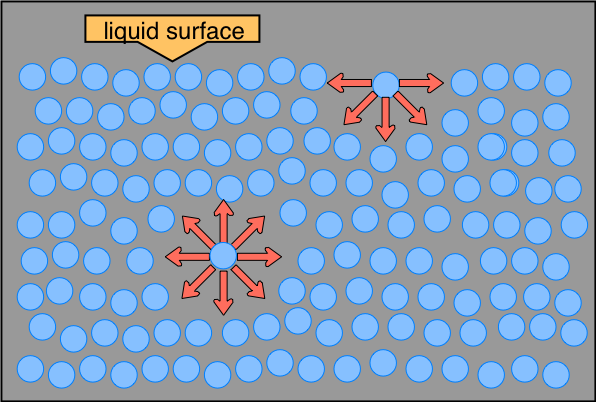Review the properties of liquids in Section 1: What is a Liquid?, in Liquids and their Interfaces.

### 5e: Discuss the process by which liquids can change into a solid or a gas.

Phase changes occur when a substance changes between different states—solid, liquid or gas.

• Define vapor pressure.
• How does vapor pressure lead to phase change for liquids?
• Define nucleation.
• How does nucleation lead to phase change for liquids?

All substances have a property known as free energy, which describes the tendency of a substance’s thermal energy to escape and disperse.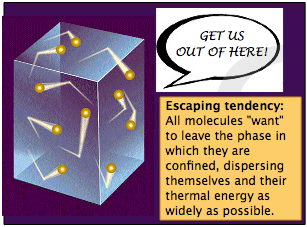Equilibrium vapor pressure, or vapor pressure, measure the tendency of surface liquid molecules to escape into the gas phase.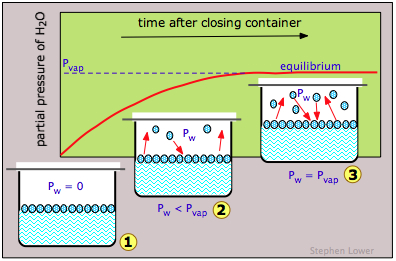In the figure above, when the container of water is open, no pressure from water molecules that have gone into the gas phase exists (as they escape into the rest of the room).

We can express this statement mathematically as Pw = 0 (i.e., the pressure of water equals zero).

When the container is closed, the pressure of the water molecules in the gas phase directly above the liquid increases (since they can no longer escape the container). Some water molecules escape to the gas phase, and some go back into the liquid phase. This builds until an equilibrium is reached among particles going into the gas phase and particles going back into the liquid phase.

When the equilibrium is reached, the system is at its equilibrium vapor pressure. For a liquid to boil, the vapor pressure must equal the external pressure.

During freezing, liquids turn into solid: the vapor pressure of the liquid equals the vapor pressure of the solid. The liquid changes phase into a solid.

### 5f: Define the characteristics of bonding in ionic compounds.

Ionic solids form a lattice of oppositely-charged ions held together by Coulombic charges.

• What types of elements are generally involved in making ionic compounds?
• How does ionic bonding differ from covalent bonding?
• What do ionic compounds look like? What are their properties?

Ionic compounds are composed of positively and negatively charged ions held together by Coulombic charges. In other words, opposite charges are attracted to each other and the ionic solids form crystal lattices with a regular, repeating structure. This is different from covalently bonded compounds which are held together by covalent bonds.

This figure displays the crystal lattice of sodium chloride (table salt):Ionic compounds are hard. Hardness measures how resistant a substance is to being deformed. Ionic compounds are also brittle. Brittleness means that one layer of the crystal lattice can “slip” over another when it is hit with a physical stress, which can cause the substance to break.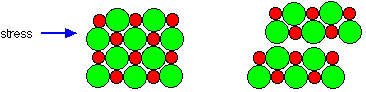For more details and examples of ionic solid structure, review Section 1: Introducing Ionic Solids, in Ionic and Ion-derived Solids.

### 5g: Interpret phase diagrams.

A phase diagram details the phase a substance will be in at any temperature and pressure.

• Identify the parts of a phase diagram, including the critical point and triple point.
• For a given temperature and pressure, determine the state of matter on a phase diagram.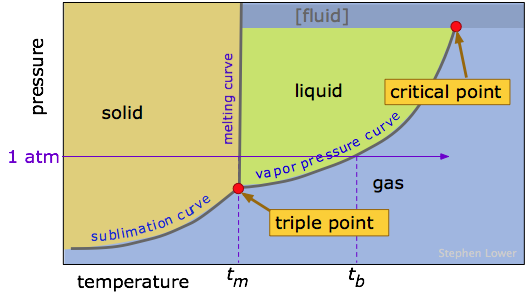This figure details a general phase diagram for a given substance.

• The horizontal axis shows the temperature, and the vertical axis shows the pressure.
• The lines or curves are phase boundaries or conditions where the substance exists in equilibrium between two phases.
• The area on the left (colored in yellow) shows the set of temperature and pressure conditions where a solid exists.
• The area in the top middle (in green) shows the set of temperature and pressure conditions where a liquid exists.
• The area in the bottom to the right (in blue) shows the set of temperature and pressure conditions where a gas exists.
• The triple point displays the point where all three states of matter (solid, liquid, and gas) are in equilibrium.
• The critical point displays the point where you can no longer have a liquid or a gas. Rather, after the critical point, a form of matter called a supercritical fluid is formed. Supercritical fluids have a unique set of properties that are similar to both liquids and gases. Supercritical fluids flow through solids like gases and can dissolve substances like liquids.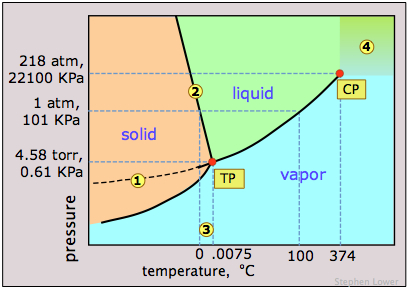This graph shows the phase diagram of water. Look at the four numbered points to determine the phase or phases of matter present at those conditions.

• At the pressure and temperature point labeled number one, the water is a solid, since the point is in the solid region of the diagram.
• At point two, the water is at a phase boundary (the liquid-solid phase boundary). This means the water is in equilibrium between its solid and liquid state.
• At point three, the water is in the gas or vapor state.
• At point four, the water is above the critical point, which means it is in the state of supercritical fluid.

Review more examples of phase diagrams in Section 3: Phase Maps, in Phases, Changes of State.

### 5f: Describe and explain the processes of boiling, evaporation, freezing, melting, and sublimation.

There are five common types of phase changes.

• Describe the processes of boiling, evaporation, freezing, melting, and sublimation.
1. Boiling describes the change from liquid to the gas phase. Boiling occurs when the vapor pressure of the liquid equals the vapor pressure of the external environment. At this point, surface liquid molecules can escape into the gas phase.

2. Evaporation occurs when the liquid container is open and the vapor pressure of the liquid is sufficient for surface molecules to escape into the gas phase.

3. Freezing occurs when the liquid particles do not have sufficient energy to remain in the mobile liquid phase. Freezing occurs when the vapor pressure of the liquid equals that of the solid.

4. Melting occurs when the solid molecules gain sufficient energy to escape into the liquid phase.

5. Sublimation occurs when the solid and gas phases are in equilibrium.

### Unit 5 Vocabulary

• Atmosphere
• Boiling
• Brittleness
• Coulombic charge
• Constant pressure
• Critical point
• Crystal lattice
• Elastic collision
• Equilibrium vapor pressure/vapor pressure
• Evaporation
• Free energy
• Freezing
• Gas constant
• Hardness
• Ideal gas
• Ideal gas equation
• Ideal gas law
• Intermolecular forces
• Ionic compound
• Kelvin
• Kinetic energy
• The Kinetic Molecular Theory of Gases
• Melting
• Mobility
• Nucleation
• Phase boundaries
• Phase change
• Phase diagram
• Pressure
• Sublimation
• Supercritical fluid
• Surface tension
• Triple point
• Vapor pressure
• Viscosity
Last modified: Wednesday, July 17, 2019, 6:06 PM